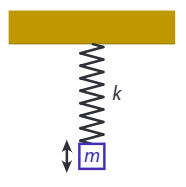$$\require{cancel}$$

# 1.2: Dimensional Analysis

Although you will of course need a complete physical model (represented as a set of mathematical equations) to fully describe a physical system, you can get surprisingly far with a simple method that requires no detailed knowledge at all. This method is known as dimensional analysis , and based on the observation in the previous section that the two sides of any physical equation have to have the same dimension. You can use this principle to qualitatively understand a system, and make predictions on how it will respond quantitatively if you change some parameter. To understand how dimensional analysis works, an example is probably the most effective - we’ll take one that is ubiquitous in classical mechanics: a mass oscillating on a spring (known as the harmonic oscillator), see Figure $$\PageIndex{1}$$.Figure $$\PageIndex{1}$$: A harmonic oscillator: a mass m suspended on a spring with spring constant k, oscillating with a frequency $$\omega$$.
1.2.1 Worked example: dimensional analysis of the harmonic oscillator

Consider the harmonic oscillator consisting of a mass of magnitude m, suspended on a spring with spring constant k. If you pull down the mass a bit and release, it will oscillate with a frequency $$\omega$$. Can we predict how this frequency will change if we double the mass?

Solution

There are two ways to answer this question. One is to consider all the forces acting on the mass, then use Newton’s second law to derive a differential equation (known as the equation of motion) for the mass, solve it, and from the solution determine what happens if we change the mass. The second is to consider the dimensions of the quantities involved. We have a mass, which has dimension of mass ($$M$$), as it is one of our basic quantities. We have a spring with spring constant k, which has dimensions of force per unit length, or mass per unit time squared:

$[k]={F \over L}={MLT^{-2} \over L}={M \over T^2} \label{1.2.1}$

Note the notation [k] for the dimension of k. For the frequency, we have $$[\omega]={1 \over T}$$. Now we know that the frequency is a function of the spring constant and the mass, and that both sides of that equation must have the same sign. Since there is no mass in the dimension of the frequency, but it exists in the dimension of both the spring constant and the mass, we know that $$\omega$$ must depend on the ratio of k and m: $$\omega \sim {k \over m}$$. Now $${[{k \over m}]}={1 \over T^2}$$, and from $$[\omega]={1 \over T}$$, we conclude that we must have

$\omega \sim \sqrt{k \over m} \label{1.2.2}$

Equation \ref{1.2.2} allows us to answer our question immediately: if we double the mass, the frequency will decrease by a factor of $$\sqrt2$$.

Note that in Equation \ref{1.2.2} I did not write an equals sign, but a ‘scales as’ sign ($$\sim$$, sometimes also written as ). That is because dimensional analysis will not tell us about any numerical factor that may appear in the expression, as those numerical factors have no unit (or, more correctly, have no dimension - they are dimensionless).

You may object that there might be another factor at play: shouldn’t gravity matter? The answer is no, as we can also quickly see from dimensional analysis. The force of gravity is given by mg, introducing another parameter g (the gravitational acceleration) with dimension $$[g]={L \over T^2}$$. Now if the frequency were to depend on g, there has to be another factor to cancel the dependence on the length, as the frequency itself is length-independent. Neither m nor k has a length-dependence in its dimension, and so they cannot ‘kill’ the $$L$$ in the dimension of $$g$$; the frequency therefore also cannot depend on $$g$$ - which we have now figured out without invoking any (differential) equations!

Above, I’ve sketched how you can use dimensional analysis to arrive at a physical scaling relation through inspection: we’ve combined the various factors to arrive at the right dimension. Such combinations are not always that easy to see, and in any case, you may wonder if you’ve correctly spotted them all. Fortunately,there is a more robust method, that we can also use to once again show that the frequency is independent of the gravitational acceleration. Suppose that in general $$\omega$$ could depend on k, m and g. The functional dependence can then be written as2

$[\omega]={[k^{\alpha}m^{\beta}g^{\gamma}]}={M \over T^2}^{\alpha}M^{\beta}{L \over T^2}^{\gamma}={M^{\alpha + \beta}T^{-2(\alpha + \gamma)}L^ \gamma}$

which leads to three equations for the exponents:

$$\alpha +\beta =0$$
$$-2(\alpha - \gamma) =-1$$
$$\gamma =0$$

which you can easily solve to find $$\alpha = {1 \over 2}$$, $$\beta = -{1 \over 2}$$, $$\gamma=0$$, which gives us Equation \ref{1.2.2}. This method3 will allow you to get dimensional relations in surprisingly many different cases, and is used by most physicists as a first line of attack when they first encounter an unknown system.

2 The actual function may of course contain multiple terms which are summed, but all those must have the same dimension. Operators like sines and exponentials must be dimensionless, as there are no dimensions of the form sin(M) or $$e^L$$. The only allowable dimensional dependencies are thus power laws.

3 The method is sometimes referred to as the Rayleigh algorithm, after John William Strutt, Lord Rayleigh (1842-1919), who applied it, among other things, to light scattering in the air. The result of Rayleigh’s analysis can be used to explain why the sky is blue.